• Classroom Activity

## The Bar Model

The bar model is used in Singapore and other countries, such as Japan and the USA, to support children in problem solving

The bar model is used in Singapore and other countries, such as Japan and the USA, to support children in problem solving. It is not a method for solving problems, but a way of revealing the mathematical structure within a problem and gaining insight and clarity as to how to solve it. It supports the transformation of real life problems into a mathematical form and can bridge the gap between concrete mathematical experiences and abstract representations. It should be preceded by and used in conjunction with a variety of representations, both concrete and pictorial, all of which contribute to children’s developing number sense. It can be used to represent problems involving the four operations, ratio and proportion. It is also useful for representing unknowns in a problem and as such can be a pre-cursor to more symbolic algebra.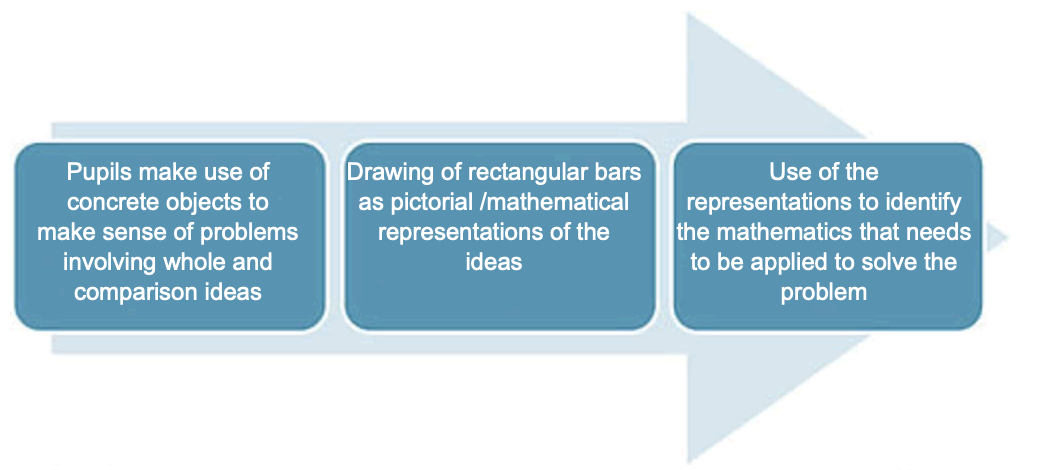The bar model supports understanding of the relationship between addition and subtraction in that both can be seen within the one representation and viewed as different ways of looking at the same relationships.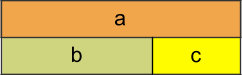This diagram encapsulates all of the following relationships;

a = b + c ; a = c + b ; a – b = c ; a – c = b

To prepare young children for the bar model it is a good idea to encourage them to line up objects in a linear arrangement when representing addition and subtraction problems.

Such arrangements will also help children to organise their counting. The physical objects can then be replaced, in time, with linking cubes and with a bar drawn next to it. The question can then be asked “what’s the same, what’s different?” to support the children in their reasoning and in making sense of the bar as an abstract representation of the physical objects. It is useful for children to work in pairs with one manipulating the cubes, while the other records by drawing the bars and then writing the number sentence underneath. The children can then swap roles.

Sam had 10 red marbles and 12 blue marbles. How many marbles did he have altogether?In problems involving addition and subtraction there are three possible unknowns as illustrated below and given the value of two of them the third can be found.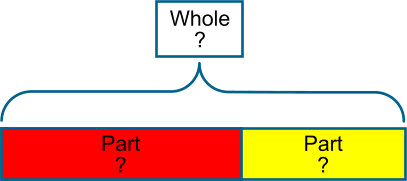The examples below illustrate a variety of ways that the bar might be used for addition and subtraction problems. A question mark is used to indicate the part that is unknown.I have 6 red pencils and 4 yellow pencils. How many pencils do I have?

(I combine two quantities to form the whole)

## AdditionAugmentation- a quantity is increased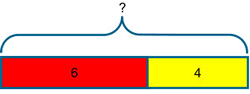I have 6 red pencils and I buy 4 yellow pencils. How many pencils do I have?

(The bar I started with increases in length)

## Subtraction- Take AwayI had 10 pencils and I gave 6 away, how many do I have now?

(This time we know the whole but only one of the parts, so the whole is partitioned and one of the parts removed to identify the missing part)

## Subtraction- Comparison or Difference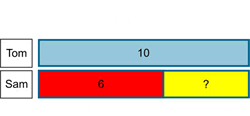Tom has 10 pencils and Sam has 6 pencils. How many more does Tom have?

(The bar is particularly valuable for seeing the difference between the two quantities)

## Equivalence

The model can be rearranged to demonstrate equivalence in a traditional layout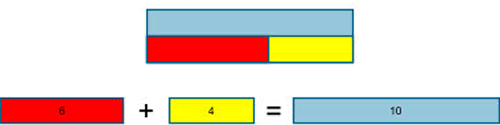Pupils need to develop fluency in using this structure to represent addition and subtraction problems in a variety of contexts using the bar model. The model will help children to see that different problems share the same mathematical structure and can be visualised in the same way. Asking children to write their own problems, using the bar as the structure will help to consolidate this understanding.

All of these concepts involve proportional and multiplicative relationships and the bar model is particularly valuable for representing these types of problems and for making the connections between these concepts visible and accessible.

### Multiplication

Notice how each section of the bars in the problem below has a value of 4 and not 1. This many-to-one correspondence, or unitising is important and occurs early, for example in the context of money, where one coin has a value of 2p for example. It is also a useful principle in the modelling of ratio problems.

Peter has 4 books
Harry has five times as many books as Peter.
How many books has Harry?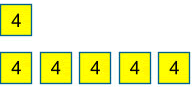4 × 5 = 20
Harry has 20 books

### Division

When using the bar model for division it is the image of sharing rather than grouping which is highlighted in this representation.

Mr Smith had a piece of wood that measured 36 cm.
He cut it into 6 equal pieces.
How long was each piece?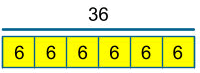36 ÷ 6 = 6
Each piece is 6 cm

### Problems Involving Proportion

When modelling problems involving proportion it is useful to divide the bar into equal parts so that the proportional relationship and multiplicative structure are exposed.

Once the value of one part is labelled, the other parts can be identified as they are the same, for example in this KS2 SATS Question.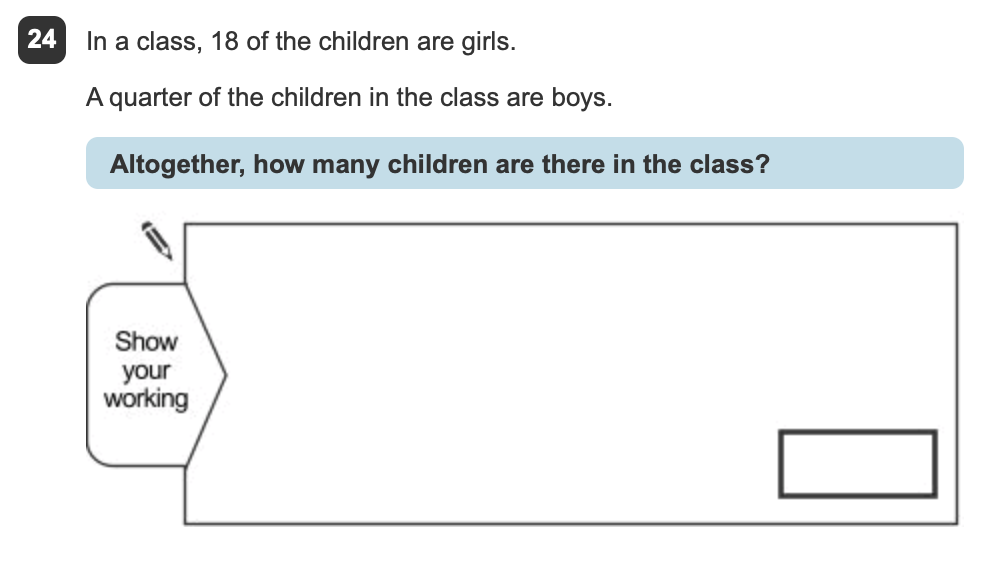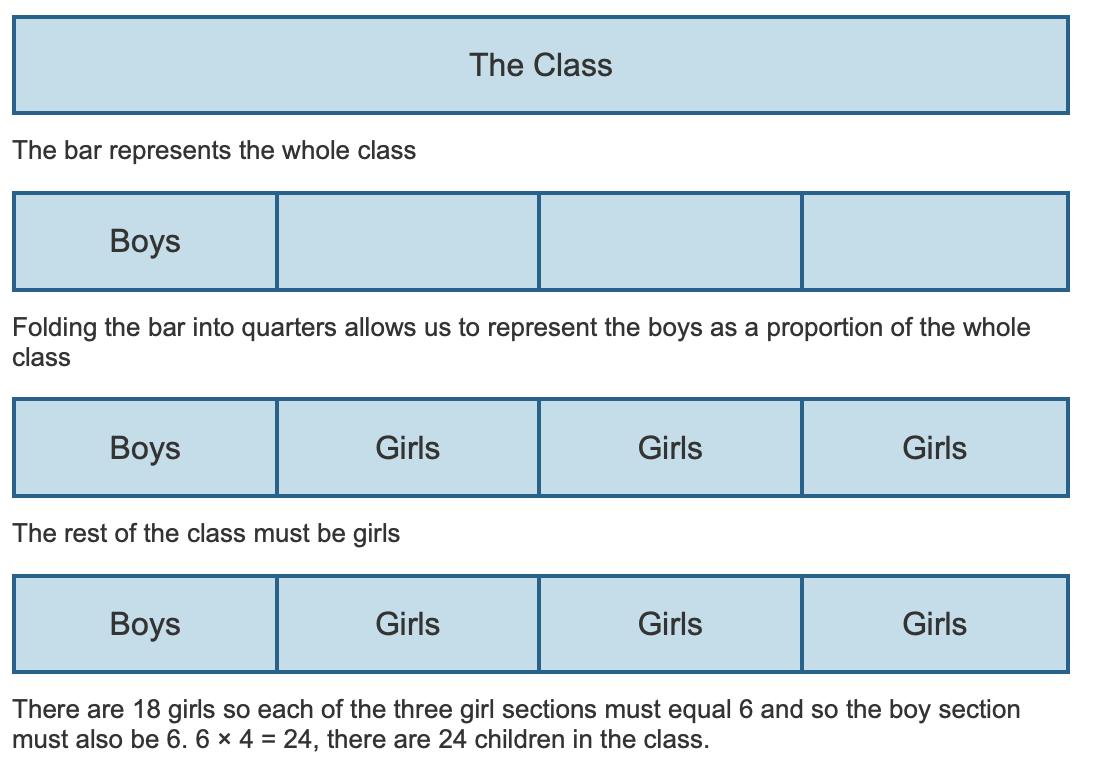### Fractions

The bar model is valuable for all sorts of problems involving fractions. An initial step would be for pupils to appreciate the bar as a whole divided into equal pieces. The number of equal pieces that the bar is divided into is defined by the denominator. To represent thirds, I divide the bar into three equal pieces, to represent fifths I divide the bar into five equal pieces. A regular routine where pupils are required to find a fraction of a number by drawing and dividing a bar, using squared paper would be a valuable activity to embed both the procedure and the concept and develop fluency.

Find 15 of 30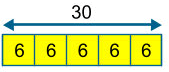The same image can be used to find 25 or 35 of 30 etc.

Finding the original cost of an item that has been reduced in a sale is one that pupils find particularly tricky. The ease at which such problems can be solved is demonstrated below:

A computer game is £24 in the sale. This is one quarter off its original price. How much did it cost before the sale?The bar represents the original cost. It is divided into quarters to show the reduced cost of £24.

£24 ÷ 3 = £8, giving the value of three sections of the bar. The final section of the bar must also be £8, since it represents the same proportion as each of the other sections.

£8 × 4 = £32

The original cost of the computer game is £32

### Percentages

Problems involving percentages are solved in a similar way to those involving fractions. The key is to divide the whole into equal parts.

A computer game is reduced in a sale by 30%. Its reduced price is £77. How much was the original price?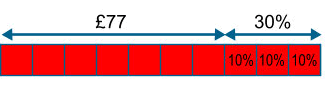Dividing the bar into ten equal pieces allows us to represent 30% and keep the other pieces the same size.

£77 ÷ 7 = £11

The original cost (the whole bar) is £11 × 10 = £110

### Problems involving Ratio

The ratio problem outlined below is represented with double sided counters. These can be used as an alternative representation to drawing a bar. The advantage of this is that they can be moved around to represent a change in relationship.

Sam and Tom have football stickers in the ratio of 2 to 3. Altogether they have 25 stickers. If Sam gives half of his stickers to Tom, how many will Tom have?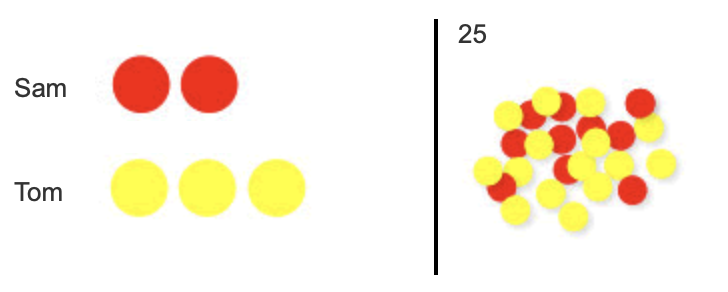Step 1

Represent the ratio

Step 2

Recognise that if together the counters have a value of 25 then one has a value of 5

Step 3

Give half of Sam's stickers to Tom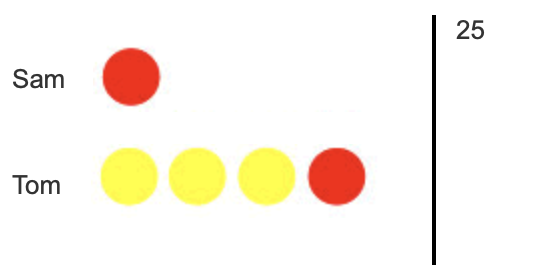Step 4

Multiply 5 by 4 to give the total value of Tom's stickers

5 × 4 = 20Notice how the many-to-one correspondence, as discussed above, allows this problem to be modelled efficiently. Children may at first use a one-to-one correspondence (i.e. each counter having a value of 1) and represent the problem using 25 counters, setting them out in a two to three ratio as illustrated below.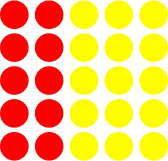Children can then be supported in transferring their understanding to many-to-one correspondence as illustrated above (i.e. that every 5 counters in each column can be replaced with one counter worth 5). The flexibility of appreciating that the value of one counter can change depending on the context and the total quantity helps to develop the pupils’ algebraic reasoning.

Notice how the strategy explored above supports this KS2 SATS questionThe bar can be a valuable representation to enable children to represent problems in such a way that the mathematical structure is exposed.

This enables pupils to ‘see’ the problem clearly and to then recognise the strategy they need to solve the problem. The appendix contains sets of problems for each key stage for you to try with the pupils you work with.

### Appendix

These problems have been tried and tested in a school for the year groups specified; however you may wish to use them more flexibly for different aged children than that specified.

#### Year 1 Problems

• 1. Ebony has 5p and Daniel has 8p. How much do they have altogether?
• 2. A lolly costs 6p. Amrit paid with a 10p coin. How much change does he get?
• 3. Michael says that 16 + 5 = 21. Is he correct?
• 4. I think of a number. I subtract 5. The answer is 4. What was my number?
• 5. How many gloves are there altogether in 6 pairs of gloves?
• 6. Twelve people are split into two groups. How any are in each group?
• 7. Mrs Morton puts five 5p coins into her purse. How much is in her purse altogether?

#### Year 2 Problems

• 1. Dylan has 37 coloured pencils and he buys 30 more. How many does he have now?
• 2. Janie has 40 beads. She loses 25 of them. How many does she have left?
• 3. What is the difference between seventy six and thirty five?
• 4. I think of a number. I subtract 5. The answer is 4. What was my number?
• 5. Last week Ellie got £1.00 pocket money. She spent half of it. How much has she got left?
• 6. A tub contains 24 coins. Saj takes 5 coins. Joss takes 10 coins. How many coins are left in the tub?
• 7. Amelia writes the calculation below as a multiplication calculation? What might she write?
3 + 3 + 3 + 3 + 3 = 15
• 8. Mr Siddique shares £18 equally between his three sons. How much does each son get?
• 10. Danny cuts his pizza into 8 equal slices. He eats 34 of the pizza and gives the rest to his dog, Gruff. How many pieces does Danny eat?

#### Year 3 Problems

• 1. There are 334 children at Springfield School and 75 at Holy Trinity Nursery. How many children are there altogether?
• 2. Gemma collected 293 badges but she gave 45 of them to her friend, Rebecca. How many badges did she have left?
• 3. Aiden has seven marbles and Harvey has fifteen. They decide to share them equally between them. How many do they get each?
• 4. Seven people each put five pens into a pot. Carmen then takes out fifteen pens. How many pens are left?
• 5. If you spend 61p at the corner shop, how much change do you get from £1.00?
• 6. If five apples cost fifty pence, how much would two apples cost?
• 7. Emma buys seven markers for 30p each. How much change does she get from £3.00?
• 8. A bookcase in the library holds 5 shelves with 46 books on each shelf. How many books are there in the bookcase altogether?
• 9. How many 5p stickers can Alexis buy with his 55p pocket money?
• 10. Which is the larger amount, one third of £60 or one quarter of £88?
• 11. A computer game is £24 in the sale. This is one quarter off its original price. How much did it cost before the sale?

#### Year 4 Problems

• 1. Martin has saved £6.78 and spends £4.69. How much does he have left?
• 2. Sally has 40 football cards. She gives 25 of them away. How many does she give away?
• 3. Sally has 30 football cards. She gives 25 of them to her friend. How many does she have left?
• 4. 8 children each download 59 songs to play on their iPod. How many songs do they have altogether?
• 5. Calculate how many fives there are in 85?
• 6. At the dressmakers, Debbie buys buttons weighing 3 grams each. If she has 81 grams of buttons, how many buttons does she buy?
• 7. Kelly buys four fifths of the shop’s oranges. If the shop had 20 oranges, how many does she have?

#### Year 5 Problems

• 1. Every day for 4 days Helen scored 7.5 in a test. On the fifth day she scored 8. What was her total score?
• 2. I cut 60 cm from 3.3m of string and shared the rest between 3 friends. How much string did they get each?
• 3. How many jugs with a capacity of 250ml could you fill with 10 litres of water?
• 4. All the children in the school are going on a residential trip to the outdoor activity centre. They will be divided into 6 equal groups If there are 246 children in the school how many will be in each group?
• 5. Robert calculated 25% of 600. What answer does he get?
• 6. Sam calculated 40% of 120. What answer does he get?
• 7. Rita worked out that 16 of a number was 12. What was the number she started with?

#### Year 6 Problems

• 1. Three quarters of a number is 54. What is the number?
• 2. Which is more; 59 of 252 or 47 of 238?
• 3. There are 36 packets of biscuits. One half are chocolate, a ninth are digestive and a third are wafer biscuits. The rest are ginger nuts. How many biscuits are ginger nuts?
• 4. There is 20% off in a sale. How much would a track suit cost, if the normal price was £44.50?
• 5. There is 20% off in a sale. The reduced price of the jeans is £36. What was the original price?
• 6. At a dance there are 4 girls to every 3 boys. There are 63 children altogether? How many girls are there?
• 7. Seven in every nine packets of crisps in a box are salt and vinegar. The rest are plain. There are 63 packets of salt and vinegar crisps. How many packets of plain crisps are there?

#### Key Stage 3 Problems

• 1. Ralph posts 40 letters, some of which are first class, and some of which are second class.
He posts four times as many second class letters as first.
How many of each class of letter does he post? (This question appeared on a GCSE higher tier paper.)
• 2. A computer game was reduced in a sale by 20% and it now costs £55 What was the original cost?
• 3. A computer game was reduced in a sale by 30% and it now costs £77. What was the original cost?
• 4. Sally had a bag of marbles. She gave one-third of them to Rebecca, and then one quarter of the remaining marbles to John. Sally then had 24 marbles left in the bag. How many marbles were in the bag to start with?
• 5. Peter is playing Space Explorer on his computer. He finished 13 of the levels last week and 25 of the remaining levels this week. He has 12 more levels to complete. How many levels does Space Explorer have?
• 6. Sam bakes a variety of biscuits.
13 are peanut, 12 are raisin, the remaining 12 were oat. How many biscuits are baked?
• 7. Tom spent 30% of his pocket money and put away 45% into his savings. He was left with £2.50. How much pocket money did he receive?
• 8. Two numbers are in the ratio 4:5. They both sum to 135. Identify both numbers.
• 9. Two numbers are in the ratio 6:7. They both sum to 169. Identify both numbers.
• 10. A herbal skin treatment uses yoghurt and honey in the ratio 5 : 3. How much yoghurt is needed to mix with 130 g of yoghurt?

• Primary
• KS1
• KS2